upm  1.7.1 Sensor/Actuator repository for libmraa (v2.0.0)
HP20X Class Reference

API for the HP20X-based Grove Barometer (High-Accuracy) More...

## Detailed Description

This is a high-accuracy barometer providing pressure, altitude, and temperature data. It can be calibrated for a given altitude offset, and a wide range of interrupt generating capabilities are supported. As usual, see the HP20X datasheet for more details.

This module was developed using a Grove Barometer (High-Accuracy) based on an HP206C chip.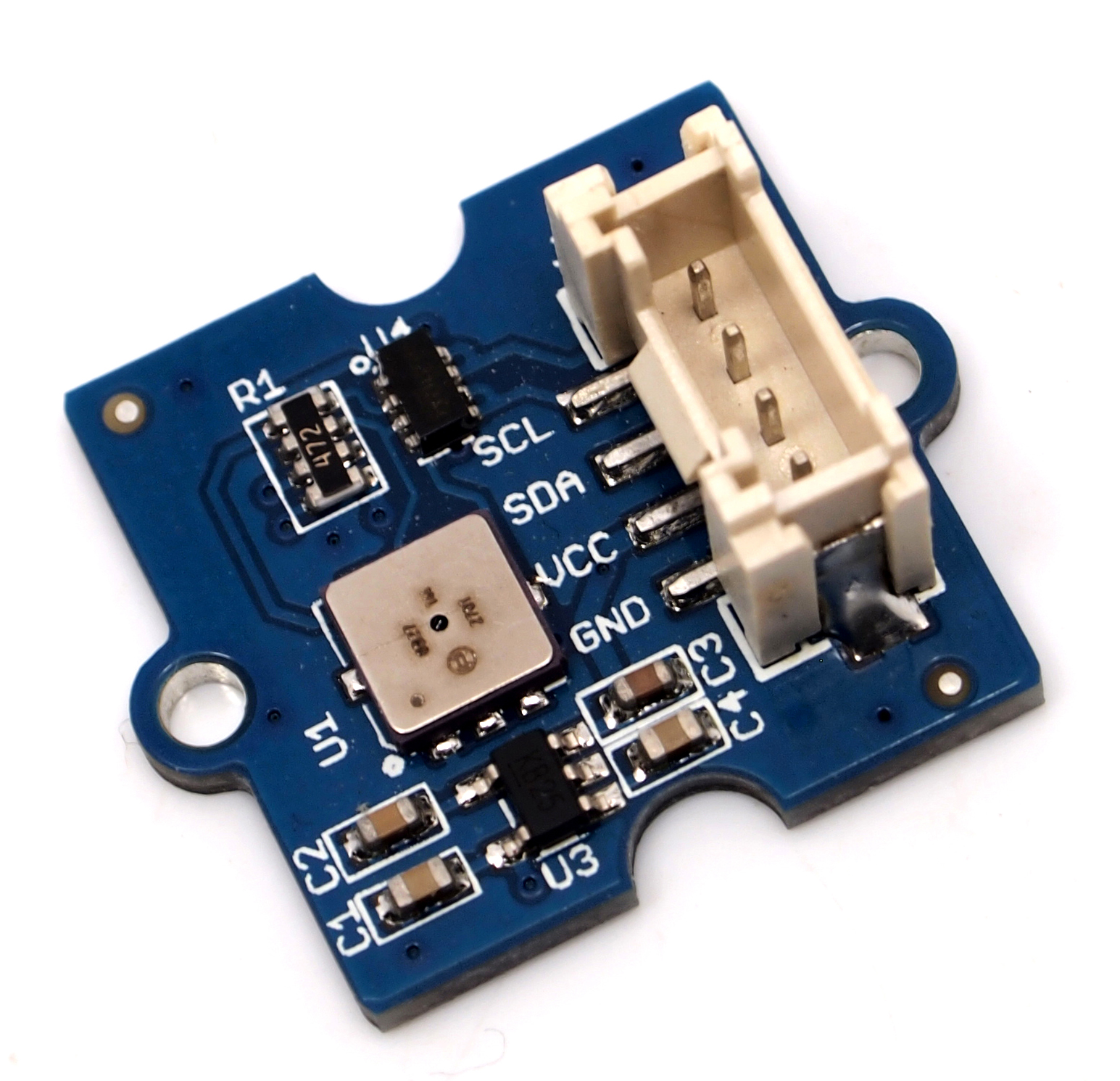// Instantiate an HP20X on default I2C bus and address
// Initialize the device with default values
bar.init();
// Output data every second until interrupted
while (shouldRun) {
printf("Temperature: %f Celsius\n", bar.getTemperature());
printf("Pressure: %f Millibars\n", bar.getPressure());
printf("Altitude: %f Meters\n", bar.getAltitude());
printf("\n");
upm_delay(1);
}

## Public Types

enum  HP20X_CMD_T {
CMD_SOFT_RST = 0x06, CMD_ADC_CVT = 0x40, CMD_READ_PT = 0x10, CMD_READ_AT = 0x11,
CMD_READ_P = 0x30, CMD_READ_A = 0x31, CMD_READ_T = 0x32, CMD_ANA_CAL = 0x28,
CMD_READ_REG = 0x80, CMD_WRITE_REG = 0xc0
}

enum  CHNL_BITS_T { CHNL_PT = 0x00, CHNL_T = 0x02, CHNL_SHIFT = 0 }

enum  DSR_BITS_T {
DSR_4096 = 0x00, DSR_2048 = 0x01, DSR_1024 = 0x02, DSR_512 = 0x03,
DSR_256 = 0x04, DSR_128 = 0x05, DSR_SHIFT = 2
}

enum  HP20X_REG_T {
REG_ALT_OFF_LSB = 0x00, REG_ALT_OFF_MSB = 0x01, REG_PA_H_TH_LSB = 0x02, REG_PA_H_TH_MSB = 0x03,
REG_PA_M_TH_LSB = 0x04, REG_PA_M_TH_MSB = 0x05, REG_PA_L_TH_LSB = 0x06, REG_PA_L_TH_MSB = 0x07,
REG_T_H_TH = 0x08, REG_T_M_TH = 0x09, REG_T_L_TH = 0x0a, REG_INT_EN = 0x0b,
REG_INT_CFG = 0x0c, REG_INT_SRC = 0x0d, REG_PARA = 0x0e
}

enum  INT_EN_BITS_T {
INT_EN_T_WIN_EN = 0x01, INT_EN_PA_WIN_EN = 0x02, INT_EN_T_TRAV_EN = 0x04, INT_EN_PA_TRAV_EN = 0x08,
INT_EN_T_RDY_EN = 0x10, INT_EN_PA_RDY_EN = 0x20
}

enum  INT_CFG_BITS_T {
INT_CFG_T_WIN_CFG = 0x01, INT_CFG_PA_WIN_CFG = 0x02, INT_CFG_T_TRAV_CFG = 0x04, INT_CFG_PA_TRAV_CFG = 0x08,
INT_CFG_T_RDY_CFG = 0x10, INT_CFG_PA_RDY_CFG = 0x20, INT_CFG_PA_MODE = 0x40
}

enum  INT_SRC_BITS_T {
INT_SRC_T_WIN = 0x01, INT_SRC_PA_WIN = 0x02, INT_SRC_T_TRAV = 0x04, INT_SRC_PA_TRAV = 0x08,
INT_SRC_T_RDY = 0x10, INT_SRC_PA_RDY = 0x20, INT_SRC_DEV_RDY = 0x40, INT_SRC_TH_ERR = 0x80
}

enum  PARA_BITS_T { PARA_CMPS_EN = 0x80 }

## Public Member Functions

~HP20X ()

bool init (DSR_BITS_T dsr=DSR_4096)

bool writeCmd (uint8_t cmd)

bool writeReg (HP20X_REG_T reg, uint8_t data)

uint8_t readReg (HP20X_REG_T reg)

float getTemperature ()

float getPressure ()

float getAltitude ()

void compensationEnable (bool enable)

bool setInterruptEnable (uint8_t bits)

bool setInterruptConfig (uint8_t bits)

uint8_t getInterruptSource ()

void setDSR (DSR_BITS_T dsr)

void recalibrateInternal ()

void softReset ()

void setAltitudeOffset (int16_t off)

void setPAThreshholds (int16_t low, int16_t med, int16_t high)

void setTemperatureThreshholds (int8_t low, int8_t med, int8_t high)

mraa::I2c m_i2c

## Member Enumeration Documentation

 enum HP20X_CMD_T

HP20X commands

 enum CHNL_BITS_T

CHNL bits

 enum DSR_BITS_T

DSR bits

 enum HP20X_REG_T

HP20X registers

 enum INT_EN_BITS_T

INT_EN bits

 enum INT_CFG_BITS_T

INT_CFG bits

 enum INT_SRC_BITS_T

INT_SRC bits

 enum PARA_BITS_T

PARA bits

## Constructor & Destructor Documentation

 HP20X ( int bus = `HP20X_I2C_BUS`, uint8_t address = `HP20X_DEFAULT_I2C_ADDR` )

HP20X constructor

Parameters
 bus I2C bus to use address Address for this device
 ~HP20X ( )

HP20X destructor

## Member Function Documentation

 bool init ( DSR_BITS_T dsr = `DSR_4096` )

Sets up initial values and starts operation

Parameters
 dsr Data sampling rate; one of the DSR_BITS_T values
Returns
True if successful

Here is the call graph for this function: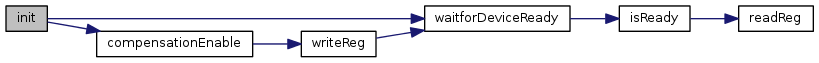bool writeCmd ( uint8_t cmd )

Sends a command to the device

Parameters
 cmd Command to send; usually, one of the HP20X_CMD_T values
Returns
True if successful

Here is the caller graph for this function: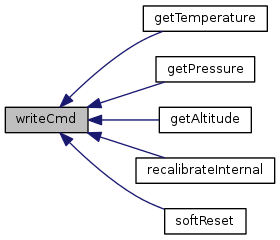bool writeReg ( HP20X_REG_T reg, uint8_t data )

Writes a value to a register

Parameters
 reg Register to write to; one of the HP20X_REG_T values data Value to write
Returns
True if successful

Here is the call graph for this function: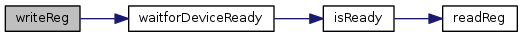Here is the caller graph for this function: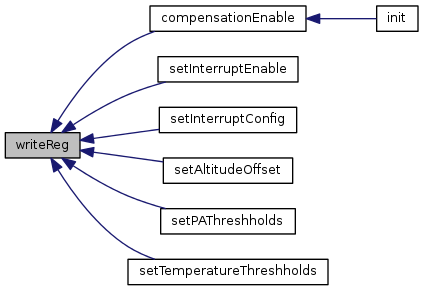uint8_t readReg ( HP20X_REG_T reg )

Reads a register and returns its value

Parameters
 reg Register to read; one of the HP20X_REG_T values
Returns
Value of a specified register

Here is the caller graph for this function: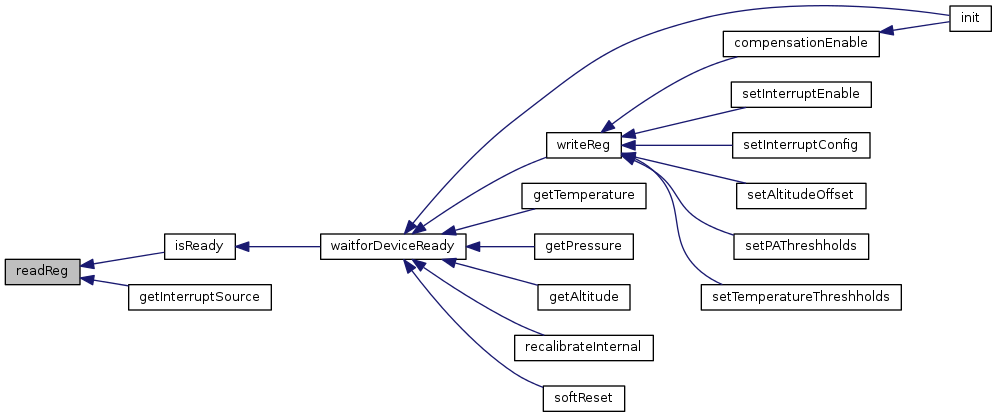int readData ( )

Reads 3 bytes of data in response to a conversion request, and converts it to an integer

Returns
Value read back (temperature, pressure, etc.)

Here is the caller graph for this function: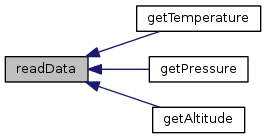bool isReady ( )

Checks to see if the DR_RDY bit is set, indicating the device can accept commands

Returns
True if the device is ready, false otherwise

Here is the call graph for this function: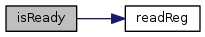Here is the caller graph for this function: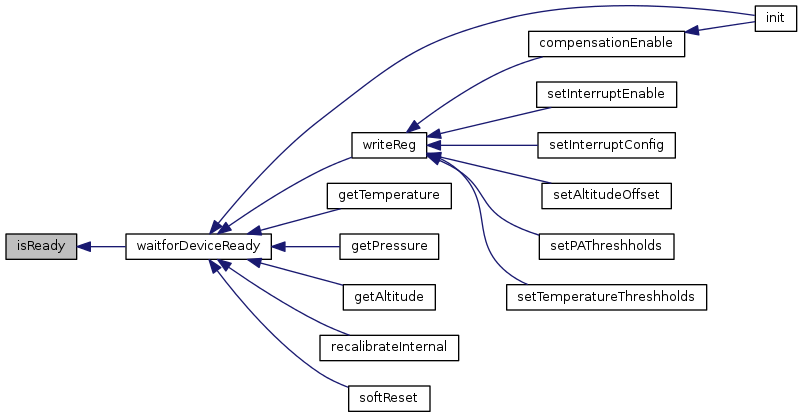bool waitforDeviceReady ( )

Checks to see if the device is ready, and sleeps/retries if not. Returns once the device indicates it's ready.

Returns
True if the device is ready; false if retries are exhausted

Here is the call graph for this function: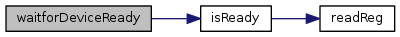Here is the caller graph for this function: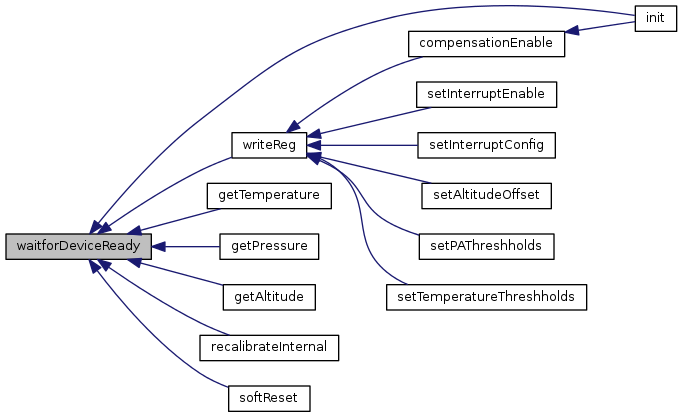float getTemperature ( void )

Returns the temperature in Celsius

Returns
Temperature

Here is the call graph for this function: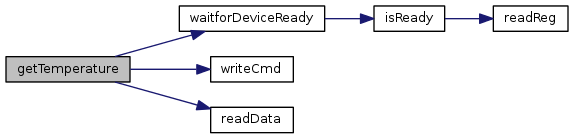float getPressure ( )

Returns the pressure in millibars

Returns
Pressure

Here is the call graph for this function: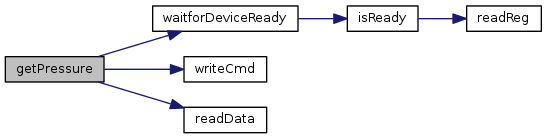float getAltitude ( )

Returns the computed altitude in meters

Returns
Altitude

Here is the call graph for this function: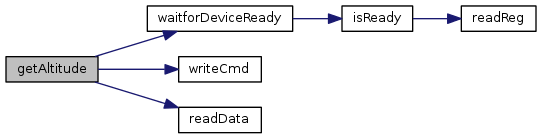void compensationEnable ( bool enable )

Enables or disables the on-chip compensator. This allows the chip to filter and clean up the output data.

Parameters
 enable True to enable, false otherwise

Here is the call graph for this function: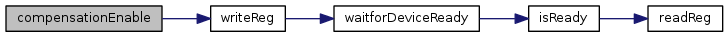Here is the caller graph for this function: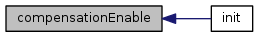bool setInterruptEnable ( uint8_t bits )

Sets up the interrupt enable register. This register defines which events can cause a hardware interrupt pin to be pulled high (active).

Parameters
 bits One or more of the INT_EN_BITS_T bits
Returns
True if successful, false otherwise

Here is the call graph for this function: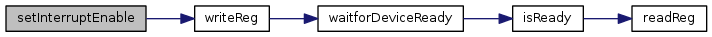bool setInterruptConfig ( uint8_t bits )

Sets up the interrupt configuration register. This register defines which events can cause an interrupt to be indicated.

Parameters
 bits One or more of the INT_EN_BITS_T bits
Returns
True if successful, false otherwise

Here is the call graph for this function: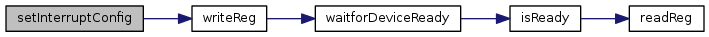uint8_t getInterruptSource ( )

Gets the interrupt source register. This register indicates which interrupts have been triggered. In addition, it indicates when certain operations have been completed.

Returns
One of more of the INT_SRC_BITS_T values

Here is the call graph for this function: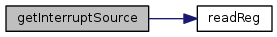void setDSR ( DSR_BITS_T dsr )

Sets the data sampling rate. Higher rates are more precise, but take more time per measurement.

Parameters
 dsr One of the DSR_BITS_T values
 void recalibrateInternal ( )

Starts an internal recalibration of analog blocks. This is faster than a soft reset.

Here is the call graph for this function: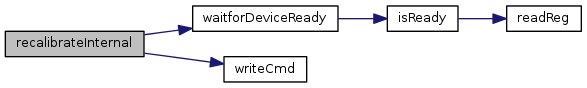void softReset ( )

Executes a soft reset. All register values are reset to power-on defaults. This function returns when the reset is complete and the device reports it is ready.

Here is the call graph for this function: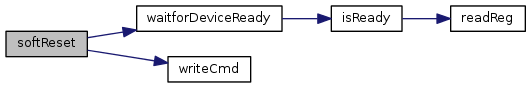void setAltitudeOffset ( int16_t off )

Sets the altitude offset for your region. See the datasheet for more details. Setting this correctly for your region is required for accurate altitude data.

Parameters
 off Offset

Here is the call graph for this function: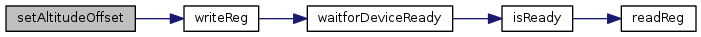void setPAThreshholds ( int16_t low, int16_t med, int16_t high )

Sets pressure/altitude thresholds for interrupt generation

Parameters
 low Low threshold to generate an interrupt med Medium threshold to generate an interrupt high High threshold to generate an interrupt

Here is the call graph for this function: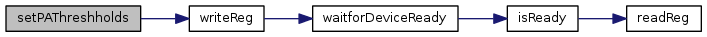void setTemperatureThreshholds ( int8_t low, int8_t med, int8_t high )

Sets temperature thresholds for interrupt generation

Parameters
 low Low threshold to generate an interrupt med Medium threshold to generate an interrupt high High threshold to generate an interrupt

Here is the call graph for this function: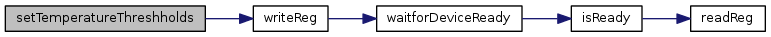The documentation for this class was generated from the following files: